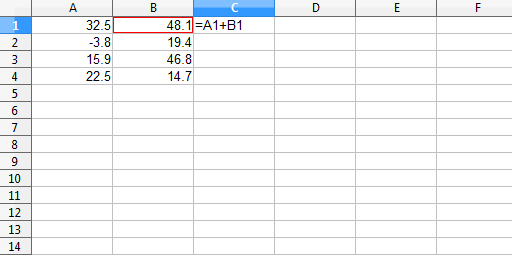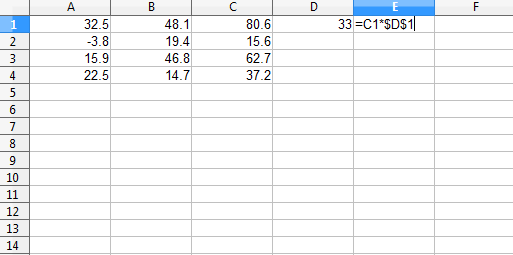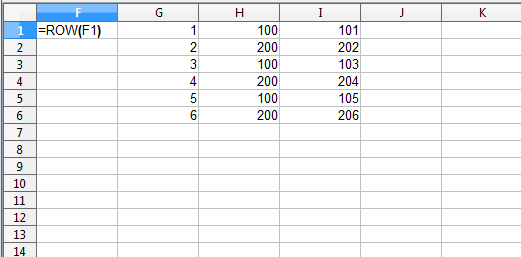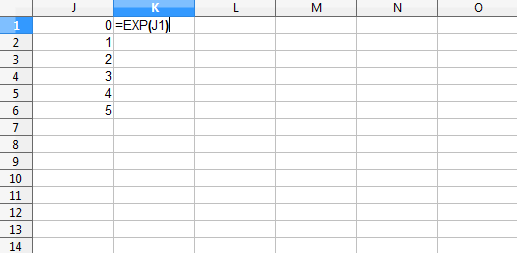# From spreadsheet thinking to R thinking

07 Jan 2014
2014/01/07

Towards the basic R mindset.

## Previously

The post “A first step towards R from spreadsheets” provides an introduction to switching from spreadsheets to R.  It also includes a list of additional posts (like this one) on the transition.

## Add two columns

Figure 1 shows some numbers in two columns and the start of adding those two columns to each other in a third column.

Figure 1: Adding two columns in a spreadsheet.The next step is to fill the addition formula down the column.

It is not so different to do the same thing in R.  First create two objects that are equivalent to the two columns in the spreadsheet:

```A <- c(32.5, -3.8, 15.9, 22.5)
B <- c(48.1, 19.4, 46.8, 14.7)```

In those commands you used the `c` function which combines objects.  You have created two vectors.  The rules for a vector are:

• it can be any length (up to a very large value)
• all the elements are of the same type — all numbers, all character strings or all logicals
• the order matters (just like it matters which row a number is in within a spreadsheet)

To summarize: they’re in little boxes and they all look just the same.

You have two R vectors holding your numbers.  Now just add them together (and assign that value into a third name):

`C <- A + B`

This addition is precisely what is done in the spreadsheet: the first value in C is the first value in A plus the first value in B, the second value in C is the second value in A plus the second value in B, and so on.

See the values in an object by typing its name:

```> C
 80.6 15.6 62.7 37.2```

The “`> `” is the R prompt, you type only what is after that: ‘`C`‘ (and the return or enter key).

Also note that R is case-sensitive — `C` and `c` are different things:

```> c
function (..., recursive = FALSE)  .Primitive("c")```

(Don’t try to make sense of what this means other than that `c` is a function.)

## Multiply by a constant

One way of multiplying a column by a constant is to multiply the values in the column by the value in a single cell.  This is illustrated in Figure 2.

Figure 2: Multiply a column times the value in a single cell, shown before filling down column E.Another way of doing the same thing is to fill the value in D1 down column D and then multiply the two columns.

Do this operation in R with:

```> C * 33
 2659.8  514.8 2069.1 1227.6```

In this command you didn’t create a new object to hold the answer.

You can think of R as doing either of the spreadsheet methods, but the fill-down image might be slightly preferable.

## Recycling in R

The R recycling rule generalizes the idea of a single value expanding to the length of the vector.  It is possible to do operations with vectors of different lengths where both have more than one element:

```> 1:6 + c(100, 200)
 101 202 103 204 105 206```

Figure 3 illustrates how R got to its answer.

Figure 3: Equivalent of the example of R’s recycling rule.Column F shows how column G was created: use the ROW function and fill it down the column.  That sequence of numbers was created in R with the ``:`` operator.

Note how the shorter vector is replicated to the length of the longer one.  Each value is used in order, and when it reaches the end it goes back to the beginning again.

You are free to think this is weird.  However, it is often useful.

## Functions

Table 1 translates between spreadsheet and R functions. The spreadsheets consulted were Excel, Works and OpenOffice. Note there is some variation between spreadsheets.

Table 1: Equivalent functions between spreadsheets and R.

 spreadsheet R comment ABS `abs` ADDRESS perhaps `assign` but there is probably a better way AND `all` more literally would be the `&` and `&&` R operators AVERAGE `mean` danger: `mean` accepts only one data argument AVG `mean` this danger of `mean` is discussed in Circle 3 of The R Inferno AVERAGEIF subscript before using `mean` BESSELI `besselI` BESSELJ `besselJ` BESSELK `besselK` BESSELY `besselY` BETADIST `pbeta` BETAINV `qbeta` BINOMDIST `pbinom` or `dbinom` `pbinom` when cumulative, `dbinom` when not CEILING `ceiling` CELL `str` is sort of the same idea CHIDIST `pchisq` `CHIDIST(x, df)` is `pchisq(x, df, lower.tail=FALSE)` CHIINV `qchisq` `CHIINV(p, df)` is `qchisq(1-p, df)` CHISQDIST `pchisq` or `dchisq` `pchisq` when cumulative, `dchisq` when not CHISQINV `qchisq` CHITEST `chisq.test` CHOOSE `switch` CLEAN `gsub` COLS ncol (Works) COLUMNS ncol (Excel, OpenOffice) COLUMN col or probably more likely `:` or `seq` COMBIN `choose` CONCATENATE `paste` CONFIDENCE CONFIDENCE(alpha, std, n) is `-qnorm(alpha/2) * std / sqrt(n)` CORREL `cor` COUNT `length` COUNTIF get length of a subscripted object COVAR `cov` CRITBINOM `qbinom` `CRITBINOM(n, p, a)` is `qbinom(a, n, p)` DELTA `all.equal` or `identical` `all.equal` allows for slight differences, and note that it does not return a logical if there’s a pertinent difference — you can wrap it in `isTRUE` if you want DGET use subscripting in R ERF see the example in `?"Normal"` ERFC see the example in `?"Normal"` EXACT `==` EXACT is specific to text, `==` is not EXP `exp` EXPONDIST `pexp` or `dexp` `pexp` when cumulative, `dexp` when not FACT `factorial` FACTDOUBLE `dfactorial` `dfactorial` is in the `phangorn` package FDIST `pf` `FDIST(x, df1, df2)` is `pf(x, df1, df2, lower.tail=FALSE)` FIND `regexpr` FINV `qf` `FINV(p, df1, df2)` is `qf(1-p, df1, df2)` FISHER `atanh` FISHERINV `tanh` FIXED `format` or `sprintf` or `formatC` FLOOR `floor` FORECAST `predict` on an `lm` object FREQUENCY you probably want to use `cut` and/or `table` FTEST `var.test` GAMMADIST `pgamma` or `dgamma` `GAMMADIST(x, a, b, TRUE)` is `pgamma(x, a, scale=b)` `GAMMADIST(x, a, b, FALSE)` is `dgamma(x, a, scale=b)` GAMMAINV `qgamma` `GAMMAINV(p, a, b)` is `qgamma(p, a, scale=b)` GAMMALN `lgamma` GAUSS GAUSS(x) is `pnorm(x) - 0.5` GCD `gcd` `gcd` is in the `schoolmath` package (and others). For more than two numbers you can do: `Reduce(gcd, numVector)` GEOMEAN `exp(mean(log(x)))` GESTEP `>=` GESTEP(x, y) is `as.numeric(x >= y)` but R often coerces automatically if needed HARMEAN `harmonic.mean` `harmonic.mean` is in the `psych` package HLOOKUP use subscripting in R HYPGEOMDIST `dhyper` `HYPGEOMDIST(x, a, b, n)` is `dhyper(x, b, n-b, a)` IF `if` or `ifelse` see Circle 3.2 of The R Inferno on `if` versus `ifelse` IFERROR `try` or `tryCatch` INDEX `[` use subscripting in R INDIRECT `get` or possibly the eval-parse-text idiom, or (better) make changes that simplify the situation INT `floor` danger: not the same as `as.integer` for negative numbers INTERCEPT (usually) the first element of `coef` of an `lm` object ISLOGICAL `is.logical` ISNUMBER `is.numeric` ISTEXT `is.character` KURT `kurtosis` `kurtosis` is in the `moments` package LARGE you can use subscripting after `sort` LCM `scm` `scm` is in the `schoolmath` package. For more than two numbers you can do: `Reduce(scm, numVector)` LEFT `substr` LEN `nchar` (Excel, OpenOffice) LENGTH `nchar` (Works) LINEST use `lm` LN log danger: the default base in R for `log` is e LOG log danger: the default base in spreadsheets for `log` is 10 LOG10 log10 LOGINV qlnorm LOGNORMDIST plnorm LOWER `tolower` MATCH `match` or `which` `match` only does exact matches. Given that MATCH demands a sorted set of values for type 1 or -1, then MATCH(x, vec, 1) is `sum(x <= vec)` and MATCH(x, vec, -1) is `sum(x >= vec)` when `vec` is sorted as MATCH assumes. MAX `max` or `pmax` `max` returns one value, `pmax` returns a vector MDETERM `det` MEDIAN median MID `substr` MIN `min` or `pmin` `min` returns one value, `pmin` returns a vector MINVERSE `solve` MMULT `%*%` MOD `%%` MODE the `table` function does the hard part. A crude approximation to MODE(x) is `as.numeric(names(which.max(table(x))))` MUNIT `diag` `diag` is much more general N `as.numeric` the correspondence is for logicals, `as.numeric` is more general NEGBINOMDIST `dnbinom` NORMDIST, NORMSDIST `pnorm` or `dnorm` `pnorm` when cumulative is true, `dnorm` when false NORMINV, NORMSINV `qnorm` NOT `!` NOW `date` or `Sys.time` OR `any` the or operators in R are `|` and `||` PEARSON `cor` PERCENTILE `quantile` PERCENTRANK similar to `ecdf` but the argument is removed from the distribution in PERCENTRANK PERMUT `function(n,k) {choose(n,k) * factorial(k)}` PERMUTATIONA PERMUTATIONA(n, k) is `n^k` PHI `dnorm` POISSON `ppois` or `dpois` `ppois` if cumulative, `dpois` if not POWER `^` PROB you can use the `Ecdf` function in the `Hmisc` package (the probabilities in the spreadsheet are the weights in `Ecdf`), then you can get the difference of that on the two limits PRODUCT `prod` PROPER see example in `?toupper` QUARTILE use `quantile` QUOTIENT `%/%` RAND runif see an introduction to random generation in R RANDBETWEEN use `sample` RANK rank RANK has the `"min"` tie.method and defaults to biggest first. `rank` only has smallest first. To get biggest first in R you can do: `length(x) + 1 - rank(x)` REPLACE `sub` or `gsub` REPT use `rep` and `paste` or `paste0` RIGHT `substring` you’ll also need `nchar` to count the characters. Alternatively you can use `str_sub` in the `stringr` package with negative limits ROUND `round` note: `round` rounds exact halves to even (which avoids bias) ROUNDDOWN `trunc` `trunc` only goes to integers ROW row or probably more likely `:` or `seq` ROWS `nrow` RSQ in `summary` of an `lm` object SEARCH `regexpr` also see `grep` SIGN `sign` SKEW skewness `skewness` is in the `moments` package SLOPE in `coef` of an `lm` object SMALL you can use subscripting after `sort` SQRT `sqrt` STANDARDIZE `scale` STD `sd` (Works) STDEV `sd` (Excel, OpenOffice) STEYX `predict` on an `lm` object STRING `format` or `sprintf` or `formatC` or `prettyNum` (Works) SUBSTITUTE `sub` or `gsub` or possibly `paste` SUM `sum` `sum` is one of the few R functions that allow multiple data arguments SUMIF subscript before using `sum` SUMPRODUCT `crossprod` TDIST `pt` TDIST(abs(x), df, tails) is pt(-abs(x), df) * tails TEXT `format` or `sprintf` or `formatC` or `prettyNum` TINV `TINV(x, df)` is `abs(qt(x/2, df))` TODAY `Sys.Date` TRANSPOSE `t` TREND `fitted` of an `lm` object TRIM `sub` TRIMMEAN `mean` `TRIMMEAN(x, tr)` is `mean(x, trim=tr/2)` TRUNC `trunc` TTEST `t.test` TYPE similar concepts in R are `typeof`, `mode`, `class`. Use `str` to understand the structure of objects UPPER `toupper` VALUE `as.numeric` VAR `var` VLOOKUP use subscripting in R WEEKDAY `weekdays` WEIBULL `pweibull` or `dweibull` `pweibull` when cumulative, `dweibull` when not ZTEST use `pnorm` on the calculated statistic

The trigonometric functions, like `cos`, `acos`, `acosh` are the same, except the R functions are all in lowercase.

### Arguments

Spreadsheets show you the arguments of a function.  The `args` function in R provides similar information.  For example:

```> args(sample)
function (x, size, replace = FALSE, prob = NULL)
NULL```

This shows that `replace` and `prob` both have default values, and so are not required.  Actually `size` is not required either — `x` is the only mandatory argument.

You will learn to not even see the `NULL` on the final line of the result of `args`.

### Help

You can get help for a function with the question mark operator:

`?sample`

This will show you the help file for the object — `sample` in this case.  It is best not to let yourself be overwhelmed by a help file.

## R vectorization

Most of the R functions are vectorized.

This is like creating a new spreadsheet column where an argument of the function is a value from the same row but a different column.  Think of putting `=EXP(A1)` in cell B1 and then filling it down.

Figure 4: EXP example of the vectorization idea, shown before column K is filled down.Giving a vector to `exp` returns the exponential of each of the values in the input vector:

```> exp(0:5)
   1.000000   2.718282   7.389056  20.085537
  54.598150 148.413159```

The result is a vector of length 6 — the same length as the input. The number in square brackets at the start of each line of output is the index number of the first item on the line.

## Some R resources

“Impatient R” provides a grounding in how to use R.

“Some hints for the R beginner” suggests additional ways to learn R.

## Epilogue

And they’re all made out of ticky tacky
And they all look just the same

from “Little Boxes” by Malvina Reynolds (1900 – 1978)

9 replies
1.anspiess says:

Nice post! I think you touched one of the most negative sides of R: the default recycling to the length of the longer vector (or column). I could guess that many errors stay unnoticed when doing column-wise summary statistics, i.e. the mean will be completely wrong if the original entry was of shorter length. I think, the default should be to fill with NA’s… and I heard many other people feel the same.

Cheers,
Andrej

Reply
2.Dominykas Grigonis says:

Thank you. Your post finally helped me to have an overview of an excel functions…

I do not really like it and I use R. At home… However at work I mostly do VBA and while it is possible to have nice repository for more frequently used functions, some excel functions are handy. Say all distributions used for hypothesis testing. I would rather use excel version that is most likely slow than code it up in vba.

Reply
3.Robert Brown says:

I think just about anything is better than Excel for complex modeling, and R is a very good alternative. However, one main problem with R is that it presents itself in linear, procedural line code. Working through a comprehensive understanding of a model with a client, such as a CFO, is very difficult.

I attempted to address these issues in my introductory tutorial “Business Case Analysis with R” (https://leanpub.com/bizanalysiswithr) by introducing the idea of using an influence diagram in parallel with communicating the flow of R logic in complex business case models.

However, the modeling application Analytica (http://www.lumina.com) actually resolves just about all of the problems with Excel and many of the remaining problems with R, namely that Analytica uses an integrated influence diagram to demonstrate the flow of logic of a model and that Analytica uses a technology called Intelligent Arrays that operates much more intuitively than R’s array system. I wrote a review for INFORMS here (http://www.incitedecisiontech.com/anareviewredirect.html).

Of course, Analytica doesn’t have anywhere near the broad array of libraries and classes that R does, and it is sold commercially (although there is a free version), but as far as communicating logic, auditing logic, and extending logic once a base model has been created, Analytica is definitely worth the time to consider.

Reply
4.Robert Brown says:

After thinking a bit more about my previous comment, I think it’s worth adding that we should always try to use the best tool available for the task/problem at hand. If data analysis is the task at hand, R is probably among the best of tools available. However, if business simulation/systems engineering is the task at hand, and those analyses require recursive time dependencies, multiple parallel threads of logic, multi-dimensional spaces that may need to be extended (both in size and number of dimensions) easily with little to no additional programming, something like Analytica is the best tool. When it comes to the graphical presentation of results, I would recommend marrying both R and Analytica together, as Analytica’s charting environment is still a little primitive (although very useful). The number and scale of quality of useful R charting packages available to produce beautiful and compelling graphics is unparalleled.

Reply

#### Trackbacks & Pingbacks

1. […] From spreadsheet thinking to R thinking […]

2. […] From spreadsheet thinking to R thinking […]

### Leave a Reply

Want to join the discussion?
Feel free to contribute!
© Copyright - Burns Statistics